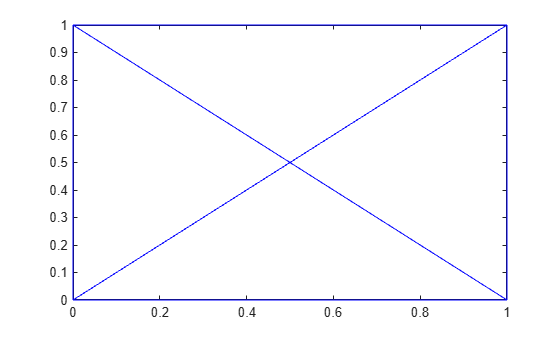edgeAttachments

Triangles or tetrahedra attached to specified edge

Description

example

ID = edgeAttachments(TR,startID,endID) identifies the triangles or tetrahedra attached to the specified edges. startID and endID specify edges by their start and end points, where each point is represented by its row number in TR.Points. The return value ID identifies triangles by their identification numbers.

example

ID = edgeAttachments(TR,E) specifies the starting and ending vertices of each edge in a two-column matrix E.

Examples

collapse all

Create and plot a Delaunay triangulation.

x = [0 1 1 0 0.5]';
y = [0 0 1 1 0.5]';
TR = delaunayTriangulation(x,y);
triplot(TR)Compute the identifications of the triangles attached to the edge defined by the starting point (0,0) and ending point (0.5,0.5). The respective vertex identifications of these points are 1 and 5.

ID = edgeAttachments(TR,1,5);
ID{:}
ans = 1×2

4     1

The ConnectivityList property indicates which vertices belong to each triangle. The fourth triangle is defined by vertices (0.5,0.5), (0,0), and (1,0), and the first triangle is defined by vertices (0,1), (0,0), and (0.5,0.5).

TR.ConnectivityList
ans = 4×3

4     1     5
4     5     3
5     2     3
5     1     2

TR.Points
ans = 5×2

0         0
1.0000         0
1.0000    1.0000
0    1.0000
0.5000    0.5000

Load 2-D triangulation data and create a triangulation representation.

TR = triangulation(tet,X);

Select two edges by their starting and ending vertex identifications.

startID = [15; 21];
endID = [936; 716];

Find the edge attachments, and examine the identifications of the triangles attached to each edge.

ID = edgeAttachments(TR,startID,endID);
ID{1}
ans = 1×6

927        2060        3438        3423        2583        4690

ID{2}
ans = 1×5

2652        3946        3953        4665        4218

Input Arguments

collapse all

Triangulation representation, specified as a scalar triangulation or delaunayTriangulation object.

Data Types: triangulation | delaunayTriangulation

Starting vertex identification for each edge, specified as a column vector. A vertex identification number is the number of the row in the Points property that corresponds to the vertex.

Data Types: double

Ending vertex identification for each edge, specified as a column vector. A vertex identification number is the number of the row in the Points property that corresponds to the vertex.

Data Types: double

Edge matrix, specified as a two-column matrix. Each row contains the starting and ending vertex identifications for an edge. A vertex identification number is the number of the row in the Points property that corresponds to the vertex.

Data Types: double